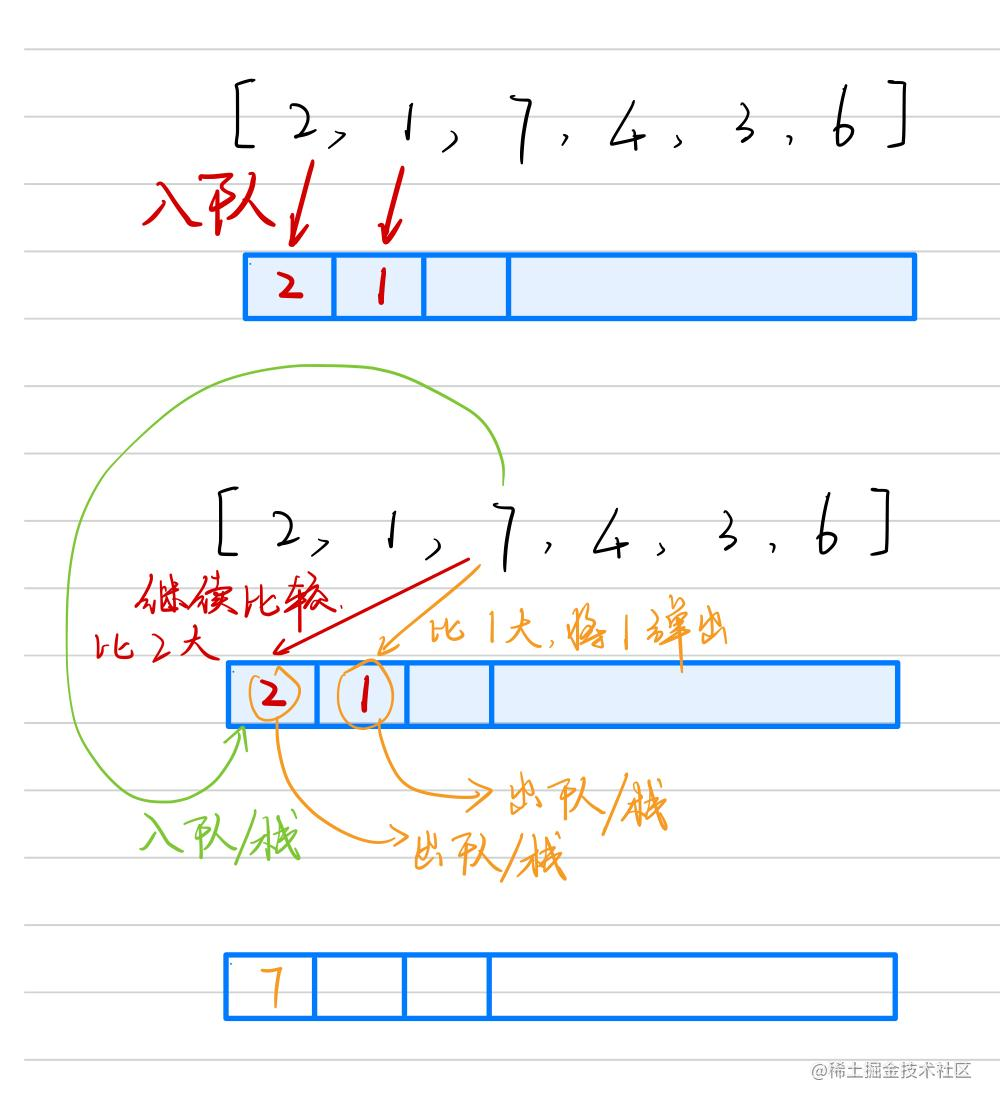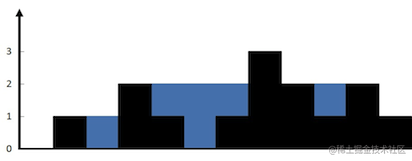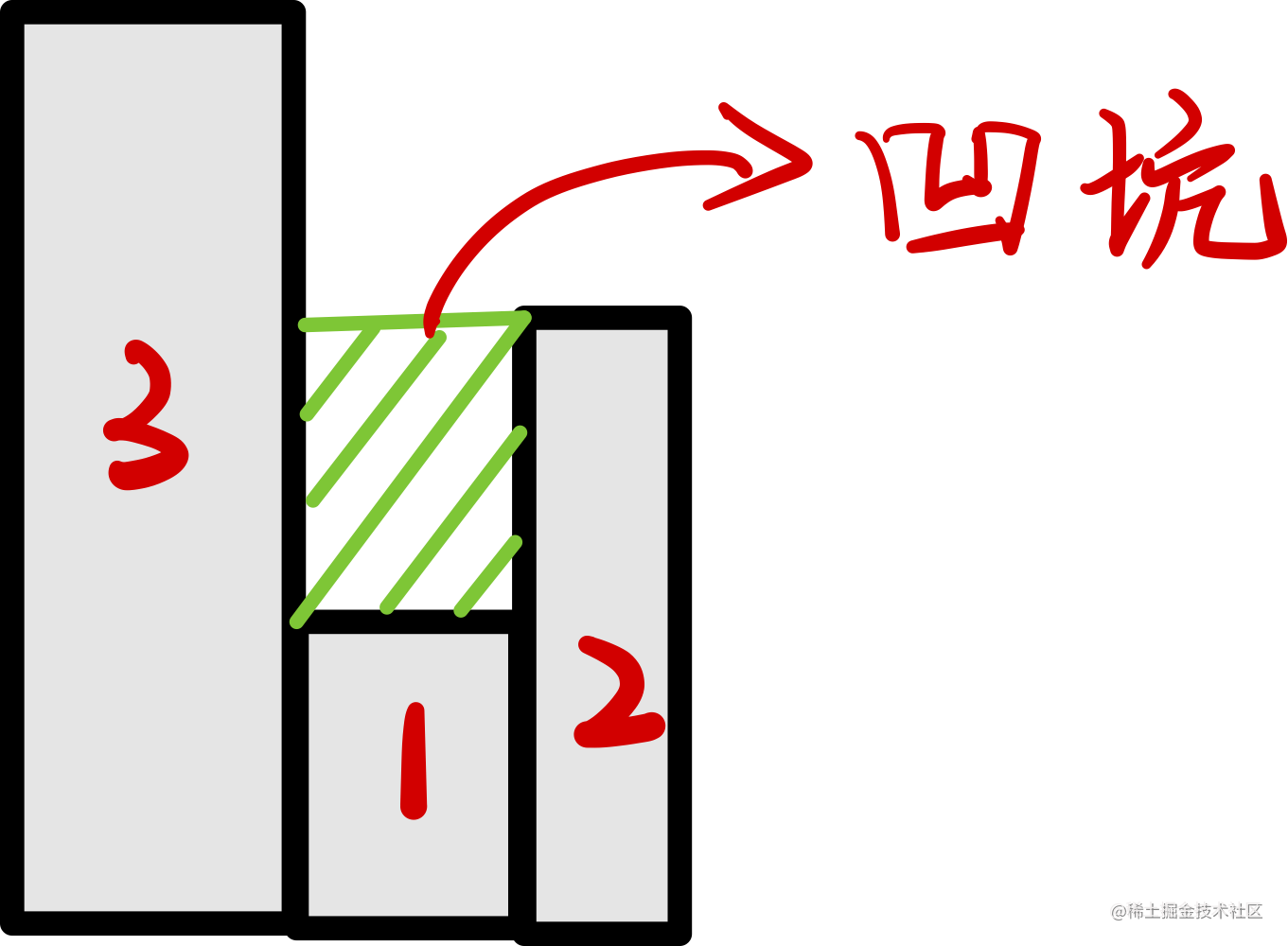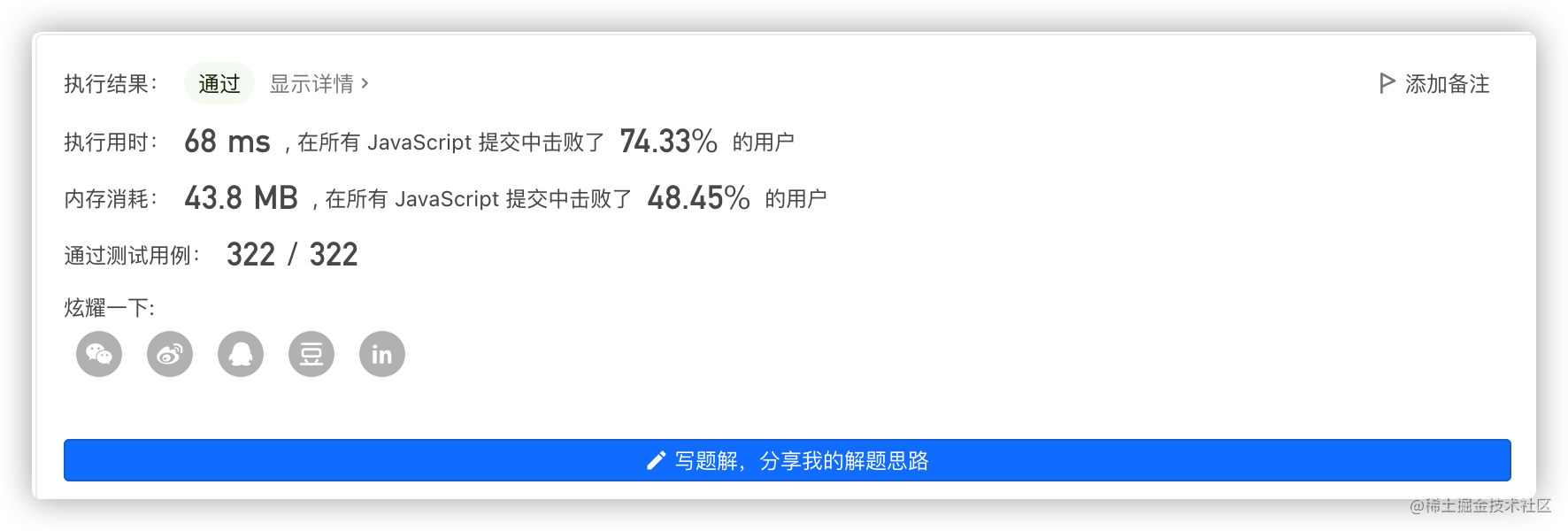# 都2022年了，你还不知道什么叫单调栈与单调队列吗？（下）1. 什么是单调栈
2. 单调栈能解决的问题
3. 单调栈经典题目的解法

# 什么是单调栈# 使用单调栈解题

## 496. 下一个更大元素 I - 力扣（LeetCode）

nums1 中数字 x 的 下一个更大元素 是指 x 在 nums2 中对应位置 右侧 的 第一个 比 x 大的元素。 给你两个 没有重复元素 的数组 nums1 和 nums2 ，下标从 0 开始计数，其中nums1 是 nums2 的子集。

4 ，用加粗斜体标识，nums2 = [1,3,4,2]。不存在下一个更大元素，所以答案是 -1 。
1 ，用加粗斜体标识，nums2 = [1,3,4,2]。下一个更大元素是 3 。
2 ，用加粗斜体标识，nums2 = [1,3,4,2]。不存在下一个更大元素，所以答案是 -1 。

1. `nums1`中的元素不一定存在于 `nums2` 数组中。
2. 我们通过将`nums2`中的元素入栈到单调栈（单调递减）中，当有元素需要被弹出时，我们就可以知道即将入队的元素就是即将被弹出元素的第一个较大的值！
3. 完成第二步时，我们需要在`nums1`中查询是否存在这个即将被弹出的元素。

``````/**
* @param {number[]} nums1
* @param {number[]} nums2
* @return {number[]}
*/
var nextGreaterElement = function(nums1, nums2) {
const top = (s) => s[s.length - 1];

const hash = {};
// 简历哈希映射，通过值来查询索引。
for (let i = 0; i < nums1.length; i++) {
hash[nums1[i]] = i;
}
const stack = [nums2];
const result = new Array(nums1.length).fill(-1);
for (let i = 1; i < nums2.length; i++) {
// 单调栈的应用，如果即将入栈的值比栈顶的数值大，就应该单调栈的规则进行弹出。
while (stack.length && nums2[i] > top(stack)) {
if (hash[top(stack)] !== void 0) {
result[hash[top(stack)]] = nums2[i];
}
stack.pop();
}
stack.push(nums2[i]);
}
return result;
};

## 42. 接雨水 - 力扣（LeetCode）1. 如果即将入栈的数值与栈顶的数值相当的话，我们应该如何处理？直接入栈处理么？还是将原来的值弹出，再将新值入栈？（其实都可以）
2. 我们在单调栈中存入的是索引值，而不是高度值，因为我们计算雨水的面积时，是通过“宽×高”的方式进行计算的，我们需要知道左边的柱子和右边柱子之间的距离。但是我们进行比较时，则仍然使用的是高度值进行比较。
``````function top(arr) {
return arr[arr.length - 1];
}
var trap = function(height) {
let sum = 0;
const stack = ;
for (let i = 1; i < height.length; i++) {

if (height[i] < height[top(stack)] {
// 即将入栈的元素比栈顶元素小，直接入栈
stack.push(i);
} else if (height[i] === height[top(stack)]) {
// 即将入栈的元素和栈顶元素相等，将原来的元素出栈，再将新元素入栈
stack.pop();
stack.push(i);
} else {
while (stack.length && height[i] > height[top(stack)]) {
// 即将入栈的元素比栈顶的元素大，向前不断递归，直到遇到栈顶元素不比新元素小位置
// “凹坑”就是栈顶的元素，
// 左边柱子则是栈顶元素下面的第一个元素
// 右边柱子则是即将入栈的元素
let mid = top(stack);
stack.pop();
if (stack.length) {
let h = Math.min(height[top(stack)], height[i]) - height[mid];
const w = i - top(stack) - 1;
sum += h * w;
}
}
stack.push(i);
}
}
return sum;
}

``````function top(arr) {
return arr[arr.length - 1];
}
var trap = function(height) {
let sum = 0;
const stack = ;
for (let i = 1; i < height.length; i++) {
while (stack.length && height[i] > height[top(stack)]) {
// 即将入栈的元素比栈顶的元素大，向前不断递归，直到遇到栈顶元素不比新元素小位置
// “凹坑”就是栈顶的元素，
// 左边柱子则是栈顶元素下面的第一个元素
// 右边柱子则是即将入栈的元素
let mid = top(stack);
stack.pop();
if (stack.length) {
let h = Math.min(height[top(stack)], height[i]) - height[mid];
const w = i - top(stack) - 1;
sum += h * w;
}
}
stack.push(i);
}
return sum;
}Accept! 搞定！